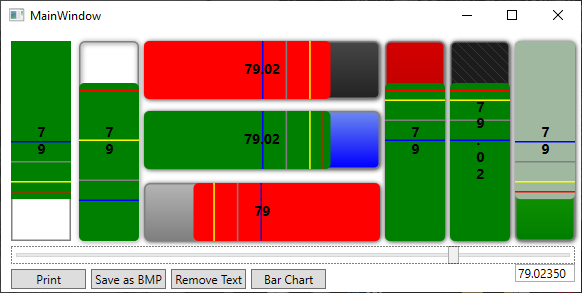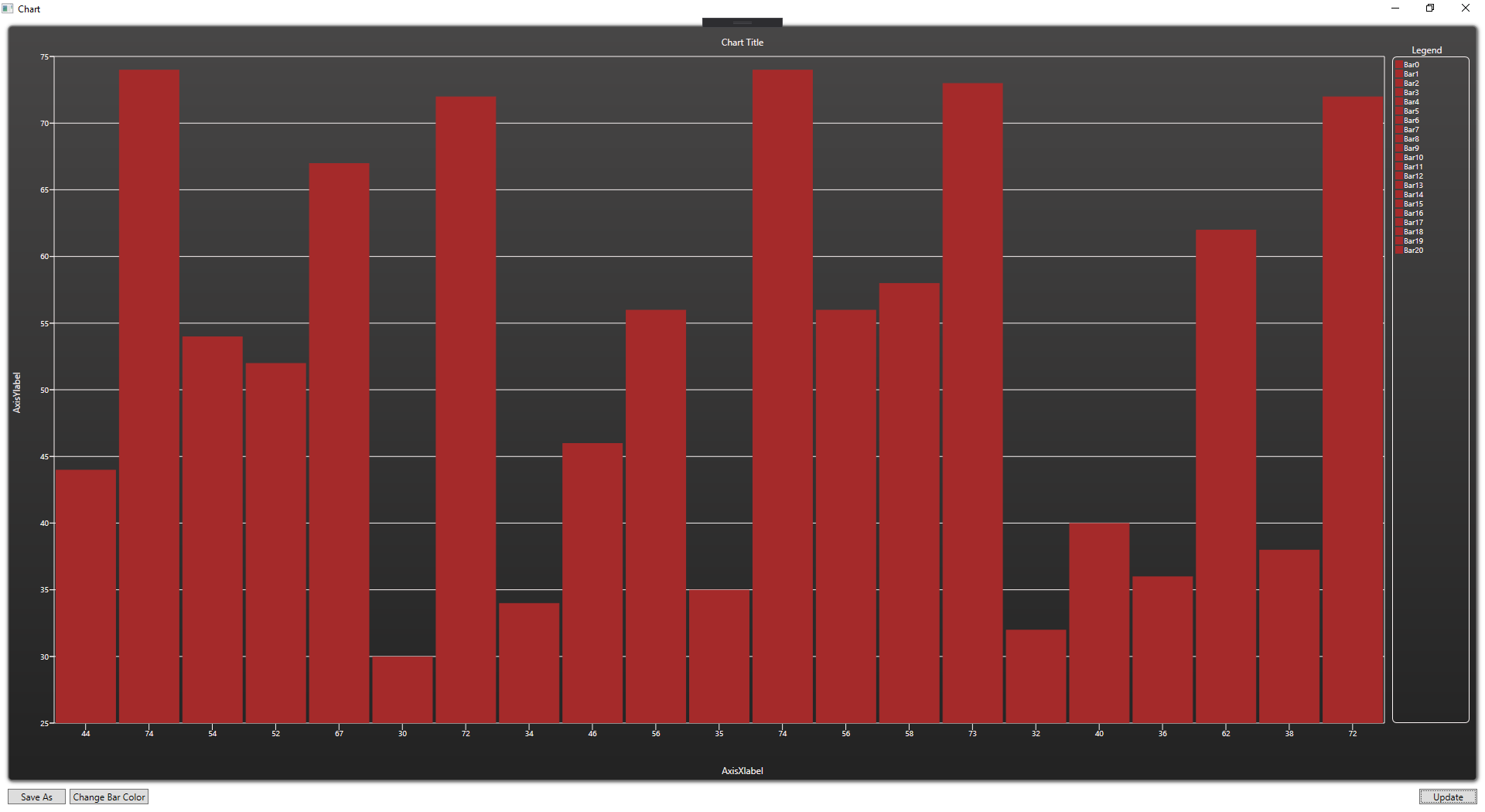HS MarkerBar and BarChartHS MarkerBar and BarChart is a Visual Studio progress-bar I built for an irrigation district SCADA system. the progress-bar needed to show high/low water values in a canal system. I also add a simple bar-chart control as well.

``````﻿Dim Value As Double = 0

Private Sub S1_ValueChanged(sender As Object, e As RoutedPropertyChangedEventArgs(Of Double)) Handles S1.ValueChanged
Value = S1.Value
Bar()
End Sub

Private Sub Bar()
Try
'Value Settings
B1.Maximum = 100
B1.Minimum = 0

'Set marker values
B1.BarMarker1Value = 75
B1.BarMarker2Value = 50
B1.BarMarker3Value = 30
B1.BarMarker4Value = 20

B1.ShowText(FormatNumber(Value, 0))
B2.ShowText(FormatNumber(Value, 2))
B3.ShowText(FormatNumber(Value, 0))
B4.ShowText(FormatNumber(Value, 2))
B5.ShowText(FormatNumber(Value, 0))
B6.ShowText(FormatNumber(Value, 2))
B7.ShowText(FormatNumber(Value, 0))
B8.ShowText(FormatNumber(Value, 0))

'Set bar value
B1.Value = Value
B2.Value = Value
B3.Value = Value
B4.Value = Value
B5.Value = Value
B6.Value = Value
B7.Value = Value
B8.Value = Value

tb1.Text = S1.Value
Catch ex As Exception
MsgBox(ex.ToString, MsgBoxStyle.Critical)
End Try
End Sub

Private Sub Button_Click(sender As Object, e As RoutedEventArgs)
'This will open a Save file dialog
B8.SaveAsBMPFileDialog()

'This one You will need to provide the path and filename EX: Image.bmp or C:\Image.bmp
'B8.SaveAsBMP("Image.bmp")
End Sub

Private Sub Button_Click_1(sender As Object, e As RoutedEventArgs)
B1.Print()
End Sub

Private Sub Button_Click_2(sender As Object, e As RoutedEventArgs)
B1.RemoveTextValue()
End Sub

Private Sub Button_Click_3(sender As Object, e As RoutedEventArgs)
Dim C1 As New Chart
C1.Show()
End Sub``````

Version: 1.5.7
.Net 4.7.2